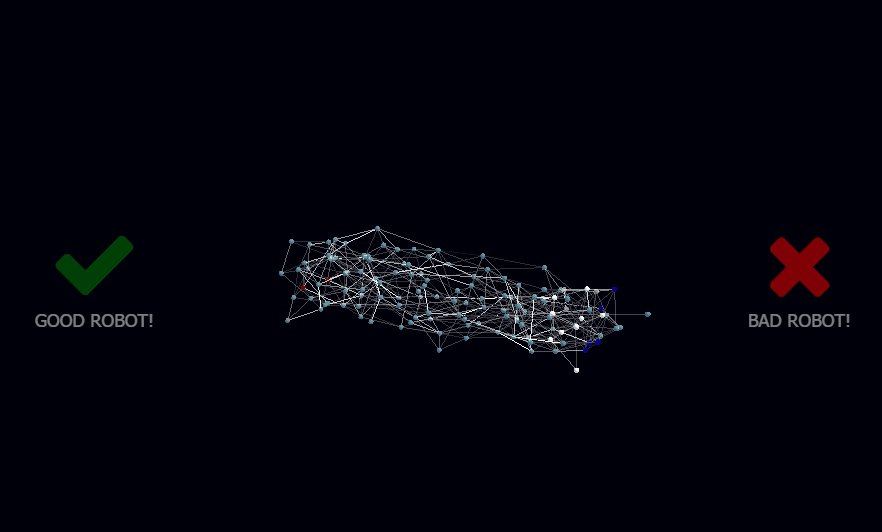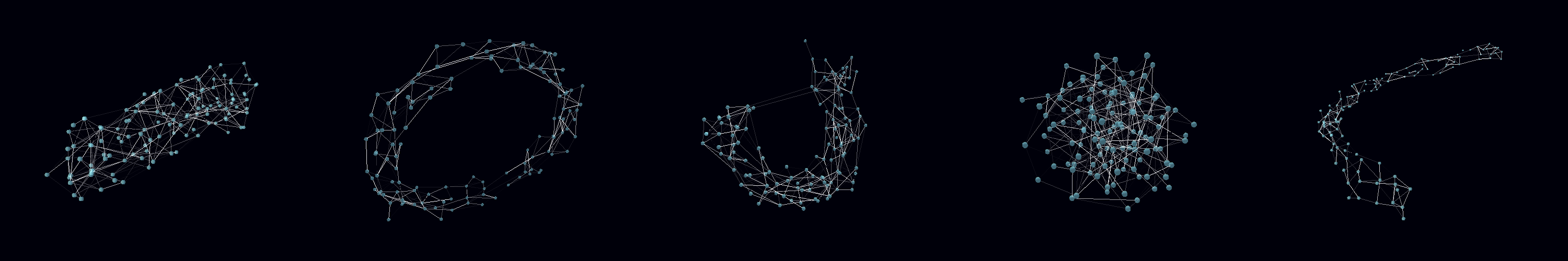# RunKit +#### Try any Node.js package right in your browser

This is a `playground` to test code. It runs a full `Node.js` environment and already has all of `npm`’s 400,000 packages pre-installed, including `botbrains` with all `npm` packages installed. Try it out:

var botbrains = require("botbrains")

This service is provided by RunKit and is not affiliated with npm, Inc or the package authors.

# botbrains v0.5.20

#### Robot brain tools to automate your nodebot# BotBrains

BotBrains is a set of artificial learning tools to automate an Arduino-based robot.

Its been built as part of an educational workshop on artificial learning specifically for International Nodebots Day.

This material here is very basic and aimed more at communicating the core concept of a neural network through practice than dealing with all the theoretical stuff that is available out there.

To read more about how this library came to be see the article on Asynchronous Neural Networks on my blog.

## Interact with your robot brains in 3D.

The key aspect of BotBrains is the ability to watch signals travel across your robot's neural network in 3D, and train it with positive and negative reinforcement.## Quick start

You need NodeJS installed, version 6 or above.

``````\$ mkdir my-bot && cd my-bot
\$ npm install botbrains
\$ cd node_modules/botbrains
\$ npm start
``````

The above will perform a quick test with a random visualization. For a full test you need to rig up a robot.

## Proper setup

You should be adding this to an existing robot project such as johnny-five.

Here is a longer example:

``````\$ mkdir my-bot && cd my-bot
\$ npm install johnny-five
\$ npm install botbrains
``````

### robot.js

``````const five = require('johnny-five');
const botbrains = require('botbrains');

const board = new five.Board({port: process.argv || '' });

const network = new botbrains.NeuralNetwork(32);

// PROXIMITY SENSOR INPUT (pin A6)
const sensor = new five.Sensor({ pin: 'A6', freq: 200 });
sensor.on('change', () => network.input('Proximity')(sensor.value / 1024));

// MOTOR OUTPUT (pins D6-D8)
const left_motor = new five.Motor({ pins: { pwm: 6, dir: 7, }, invertPWM: true, });
const right_motor = new five.Motor({ pins: { pwm: 9, dir: 8, }, invertPWM: true, });

// Output binding can be reasonably random.
// It doesn't matter what gets mapped to what
// since the robot will learn to coordinate itself
// using positive and negative feedback.

network.output('Wheel (L)')
.on('data', (power) => { // between 0 and 1
const speed = Math.floor(power * 255);
if (power > 0.25) left_motor.forward(speed);
else left_motor.stop();
});

network.output('Wheel (R)')
.on('data', (power) => { // between 0 and 1
const speed = Math.floor(power * 255);
if (power > 0.25) right_motor.forward(speed);
else right_motor.stop();
});

// DISPLAY VIA LOCAHOST (http.Server)
const server = botbrains.Toolkit.visualise(network);

});
``````

Then run it!

``````\$ node robot.js
``````

## API :: NeuralNetwork

NeuralNetwork is a class in the botbrains module and can be loaded in the following ways:

``````import { NeuralNetwork } from 'botbrains'; // ES6

const NeuralNetwork = require('botbrains').NeuralNetwork; // Node, CommonJS

const NeuralNetwork = (window || this).botbrains.NeuralNetwork; // Browser, in global context
``````

### new NeuralNetwork(size, opts)

Generates a neural network.

• `size`: The number of neurons in the neural network
• `opts`: A map of settings for the network
• `.shape`: Choice of 'drum', 'tube', 'ring', 'ball', 'sausage', 'snake', or any other in NetworkShaper.js. Defaults to 'drum'.
• `.shaper`: The shaper function used for giving shape to the network. If available will ignore `.shape`.
• `.connectionsPerNeuron`: Average synapses per neuron. Defaults to `4`.
• `.signalFireThreshold`: Threshold (between 0 and 1) needed to trigger onward neurons. Defaults to `0.3`.
• `.signalSpeed`: Speed in neurons per second. Defaults to `20`.
• `.startingWeight`: Starting weight per synapse. Defaults to `signalFireThreshold(/3 x connectionsPerNeuron)`.
• `.neuronRecovery`: Neuron recovery as fraction of `signalSpeed`. Defaults to `1/5`.
• `.learningRate`: Max increase/decrease to connection strength when learning. Defaults to `0.05`.
• `.learningPeriod`: Milliseconds in the past on which learning applies. Defaults to `20 * 1000` ms.

For example, to create a network of 100 neurons using all default options:

``````let network = new NeuralNetwork(100);
``````

To create a ring-shaped network of 100 neurons with double the speed and learning rate.

``````let network = new NeuralNetwork(1000, { shape: 'ring', signalSpeed: 40, learningRate: 0.3 });
``````

If a `String` is passed in as the `opts` parameter, its interpreted as the network shape.

``````let network = new NeuralNetwork(100, 'ring');
``````

If a `Function` is passed as the `opts` parameter, its interpreted as the shaper function, see examples in NetworkShaper.js.

``````let network = new NeuralNetwork(100, (count) => Math.floor(Math.random() * count));
``````

### network.input(label [, neurons=1])

Creates an input into the network.

• `label`: A label used to visually identify the neurons involved.
• `neurons`: Optional `Number` of neurons involved, or array of numbers (`Number[]`) defining the network nodes that are involved in the input.

Returns:

• `Function(signal 0-1)`: A function that accepts incoming signals (a float between 0 and 1).

Usage:

``````var input1 = network.input('Microphone') // 1 x Neuron assigned automatically
sensor.on('data', data => input1(data / 1024));

var input2 = network.input('LightSensor (L)', 3) // How many neurons? => 3
sensor.on('data', data => input2(data / 1024));

var input3 = network.input('LightSensor (R)', [10,11,12]) // Which neurons? => 10, 11 & 12
sensor.on('data', data => input3(data / 1024));
``````

### network.output(label [, neurons=1])

Returns an EventEmitter with 2 events: `data` and `change`.

• `label`: A label used to visually identify the neurons involved.
• `neurons`: Optional `Number` of neurons involved, or array of numbers (`Number[]`) defining the network nodes that are involved in the input.

Event: `data`:

Fires whenever there is data to output.

The event handler function will receive the following arguments.

• `data`: A numeric floating point value between 0 and 1, containing the strength of the outgoing signal.
``````network.output('Motor (L)') // 1 x Neuron assigned automatically
.on('data', (pwr) => console.log(`Power: \${pwr}.`));

network.output('Motor (R)', 4) // How many neurons? => 3
.on('data', (pwr) => console.log(`Power: \${pwr}.`));

network.output('Buzzer', [6,7,8]) // Which neurons? => 6, 7 & 8
.on('data', (pwr) => console.log(`Power: \${pwr}.`));
``````

Event: `change`:

An event that fires whenever there is a change in the outgoing data.

The event handler function will receive the following arguments.

• `data`: A numeric floating point value between 0 and 1, containing the strength of the outgoing signal.
• `last`: The previous value output.
• `diff`: The difference between `last` and `data`.
``````network.output('Buzzer')
.on('change', function(data, last, diff) {
console.log(`Buzzer output is: \${data}. Previous output was \${last}. Difference is \${diff}`);
});
``````

### network.join(network, at, surfaceArea)

Merges a network into another network, or into itself.

``````const network = new NeuralNetwork(100, 'ball');
network.join(new NeuralNetwork(100, 'ring'), 0.5, 0.1); // join on the middle, using 10% of nodes.
network.join(network); // join on itself
``````

### Shaper Function

A shaper is a function that determines the shape of the network by returning the onward connections made by each neuron.

For example, if a neuron is connected to other neurons at random, the final shape of the network will be a ball. If its connected to nearby neurons the shape will be more of a snake or cylinder. If neurons close to the end are linked to neurons at the beginning, the end product will be more of a ring or a doughnut.

The shaper function is executed once for every synapse in the network. If there are 10 nodes, and 4 synapses per node, it will fire 40 times to determine the onward neuron in each of those synapses.A shaper function has the following inputs:

• `count`: The total number of nodes in the network. In other words, in a network of 10 neurons, this will be `10`. Useful for linking up the end of the network back to its beginning or for discarding links outside the network.
• `index`: The position of the originating neuron inside the `nodes` array. In a network of 10 nodes, the first node is `0`, the last node is `9`, so a value of `9` means the last neuron in the network.
• `connectionCount`: A neuron has several connections (synapses) originating from it. For example if the neuron has 4 connections, this will be 4.
• `connectionIndex`: The position in the array of the connection currently being made. In other words, if there are 4 connections (synapses) in the originating neuron, the shaper function will execute 4 times for it, with a `connectionIndex` value of `0` to `3` accordingly.

And returns:

• `target`: The destination position of an onward neuron (for connecting to it). In a network of 10 nodes, when we are mapping a neuron `index` of `0` (the first node) and `connectionIndex` of `0` (the first synapse), a `target` of `9` means that connection is made towards the last neuron.

Bear in mind that since the shaper function will be executed multiple times per neuron to determine the onward neuron for each connection. You will want these to connect to different onward neurons. This can be done either choosing an onward `target` at random, or by using the `synapseIndex` argument to calculate the `target` neuron in a deterministic manner.

Here is an example of simple shaper function:

``````// Random ball shape
new NeuralNetwork(100, (count, index) => Math.floor(Math.random() * count));
``````

Another more involved example:

``````// Ring shape
const network = new NeuralNetwork(100, ring);

function ring(count, index) {
const width = count / 12;
const forwardBias = Math.ceil(width * Math.random());
const target = index + forwardBias;
if (target < count) {
return target;
}
return target - count; // link to beginning
}
``````

There are more examples in NetworkShaper.js.

Why does shape matter?

The Neural Network model used for botbrains is asynchronous. Signal propagate across the network in the same manner as they would in an animal brain, one neuron at a time. Different shapes matter because they create resonance and oscillation patterns that are important for producing particular outputs to inputs in a time-dependent manner.